# Performance Optimization, Prediction and Adequacy by Response Surfaces Methodology with Allusion to DRF Technique

Last Updated on 11/03/2021

### Performance Optimization, Prediction and Adequacy by Response Surfaces Methodology with Allusion to DRF Technique

Dr. Lokesh Shukla1 & Dr. Anita Nishkam2
Govt. Central Textile Institute, Kanpur1
Director, Apollo Institute of Engineering and Technology, Kanpur2
Email: drshukla_kanpur@rediffmail.com1

ABSTRACT
The RSM introduces statistically designed experiments for the purpose of making inferences from data. The second-order model is the most-frequently used approximating polynomial model in RSM. The most common designs for the second-order model are the 3k factorial, Doehlert, Box–Behnken and CCD. In this Box and Behnken Design of three variables is selected as a representative of RSM and 70:30 polyester-wool DRF yarn knitted fabrics samples as a process representative.

The survey reveals that second-order model is the most-frequently used approximating polynomial model in RSM. The Box–Behnken is most suited design for optimization and prediction of data in textile manufacturing and this model is well-suited for DRF technique yarn knitted fabric.

The trend was as higher wool fiber length shows higher, fabric weight, abrasion and bursting strength, correlation of TM was not visible however role of strands spacing is found dominant in comparison to other variables, at 14 mm spacing shows optimum behaviors.

The optimum were; weight(gms/mt2) 206 at length 75 mm, TM 2.5 and 14 mm spacing, abrasion(cycles) 1325 at length 70 mm, TM 2.25 and 14 mm spacing and bursting(kg/cm2) 14.35 at length 70 mm, TM 2.00 and 18 mm spacing.

The all selected variables fiber length, TM and strand spacing have substantial influence. The adequacies of response surface equations are very high.  The line trends of knitted fabric basic characteristics were almost same for actual and predicted models. The difference (%) was in range of 1.21 to -1.45, 2.01 to -7.26   and 17.84 to -6.61, the accuracy (%) was in range of 101.45 to 98.79, 107.27 to 97.99 and 106.61 to 82.16 and the Discrepancy Factor (R-Factor) was noted 0.016, 0.002 and 0.229 for weight, abrasion and bursting respectively between actual and predicted data’s. The L-estimation factors for actual and predicted data were i) ratio were in range of 1.01 to 0.99, 1.02 to o.93 and 1.22 to 0.94 for weight, abrasion and bursting respectively, ii) The multiple-ratio was in range of 1.26 to 0.86, iii) The ratio product was in range of 1.22 to 0.92, iv) The toting ratio was in range of 1.02 to 0.94.

KEY WORDS: Response Surface Methodology, 3k Factorial, Doehlert, Box–Behnken, Central Composite Designs, Difference (%), Accuracy (%), Discrepancy Factor (R-Factor), L-estimation Factors, Multiple-ratio, Ratio Product and Toting Ratio.

1.1 Introduction
The Response surface methodology (RSM) introduces statistically designed experiments for the purpose of making inferences from data. To achieve this goal, statistical considerations for preliminary planning of experiments, standard statistical designs for experiments and underlying logic for using these designs are emphasized. It is a common but major error to view statistics as a tool to be used only after the experiments are completed. Even using their most sophisticated tools, researchers receive data from improperly designed experiments can make only indistinct and approximate inferences. Therefore it is unfortunate, because experimental data represent an expenditure of both time and money .

In general, the theoretical model that relates some controllable variables to a response either is not available or is very complex. Identifying and fitting from experimental data an appropriate response surface model requires some use of statistical experimental design fundamentals, regression modeling techniques and optimization methods. As an important subject in the statistical design of experiments, RSM, introduced by Box and Wilson , comprises a group of mathematical and statistical techniques that is useful for empirical model building and analysis of problems in which a response of interest is influenced by several variables . It is important to fit a mathematical model equation in order to approximate a relationship between response and independent variables and determine the optimum settings of these variables that result in the maximum response.

The two important models are commonly used in RSM, including the first-order model (Equation 1) and second-order model (Equation 2) .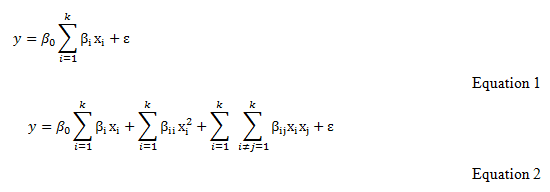Where y is the response, β0 is the constant, βi is the slope or linear effect of the factor Xi, βii is the quadratic effect of the factor xi, βij is the interaction effect between the input factors Xi and Xj and ε is the residual term. The first-order models are inadequate to represent true functional relationships with independent variables. The second-order model is most suitable, highly structured, flexible and diversified in order to locate the optimum point.

1.2 Designs for Fitting the Second-order Model
The second-order model is the most-frequently used approximating polynomial model in RSM. The most common designs for the second-order model are the 3k factorial, Doehlert, Box–Behnken and central composite designs (CCDs) [5.6]. These symmetrical designs differ from one another with respect to their selection of experimental points, number of levels for variables and number of runs and blocks.

1.3 3k Factorial Design
The 3k factorial design consists of all the combinations of the levels of the control variables, three levels each: low, medium or centre and high . The number of experimental runs (N) required for this design is defined as N = 3k, where k is the number of factors. The 3k factorial design needs a large number of experimental runs for large k, which lose its efficiency in the modeling of quadratic functions. Therefore, a 3k factorial design is more appropriate having factors numbering less than five. Due to its requirement for more experimental runs it can usually be accommodated in practice, designs that present a smaller number of experimental points, such as Doehlert, Box–Behnken and CCDs, are more often used. The application of 3k factorial design is not frequent, and the use of this design has been limited to the optimization of two variables, because its efficiency is very low for a higher number of variables.

1.4 Doehlert Design
The Doehlert (or uniform shell) design has been developed by Doehlert . The Doehlert design is for heterogeneous levels of variable. This property is important when some variables are subject to restrictions, such as cost and/or instrumental constraints or when it is important to study a variable at major or minor levels. The intervals between each variable level must have a uniform distribution . The number of experiments required (N) for the Doehlert design is defined as N = k2 + k + C0, where k is the number of factors and C0 is the number of centre points. For two variables, a central point surrounded by six points from a regular hexagon represents this design. For three variables, it is represented by a geometrical solid called a cub octahedron, and depending on how this solid is projected in the plane, it can generate some different experimental matrices. Although its matrices are neither orthogonal nor rotatable, it presents some advantages, such as requiring few experimental points for its application and high efficiency .

1.5 Box–Behnken Design
This design was developed by Box and Behnken . The Box–Behnken design provides three levels (-1, 0, +1) for each variable, which are equally spaced. The number of experiments required (N) is given by N = 2k(k -1) + C0, where k is the number of variables and C0 is the number of central points. The design is represented as a cube and all points lie on a sphere of radius √ 2. In addition, this design does not contain any points at the vertices of the cubic region created by the upper and lower limits for each variable . The Box–Behnken design for three variables takes optimization with its 13 experimental points. This design is more economical and efficient in terms of the number of required runs than their corresponding 3k designs with 27 experiments. Therefore, this design is useful in avoiding experiments that would be performed under extreme conditions, for which unsatisfactory results might occur. However, it is ineffective for situations in which we would like to know the responses at extremes.

The Box–Behnken design has been used for finding the optimum experimental conditions, leading to an optimal efficiency of different processes.

1.6 Central Composite Design
The CCD presented by Box and Wilson in 1951  is the design most commonly used for fitting second-order models and it has been subjected to much attention in the theoretical development of its properties as in its practical use . This design combines a two-level full or fractional factorial design with additional start points and at least one point at the centre of the experimental region. The CCD is widely used for the optimization of three variables. This design requires an experiment number according to N = 2k + 2k + C0, where k is the number of factors and C0 is the number of central points. In CCD, all factors are studied in five levels. This N experiment is distributed as follows [4,10].

1. Full (or fractional) 2k factorial experiments, whose factors levels are coded as −1, +1. These experiments are the only points that contribute to the estimation of the two-factor interactions.
2. Axial (or star) 2k experiments with coordinates (±α, 0, . . ., 0), (0,±α, . . ., 0), . . ., (0, 0, . . .,±α). The codified value of α is defined as α = (2k )1/4. The axial points do not contribute to the estimation of interaction terms. If curvature is found in the system, the addition of axial points allows for efficient estimation of the pure quadratic terms.
3. C0 central points at (0, 0,…, 0). These experiments provide an estimation of pure error and contribute to the estimation of quadratic terms. The CCD is a rotatable and orthogonal design. A design is rotatable if the precision of the response estimation in all directions is equal and the orthogonality of the design means that different variable effects can be estimated independently. This design has been widely used for the optimization of several processes .

1.7 Optimization by Response Surface Methodology
In most production processes, the theoretical model that relates some controllable variables (factors) to a response either is not available or is very complex. In conventional methods used to determine this relationship, experiments are carried out varying systematically the studied parameter and keeping the others constant. This should be repeated for all the influencing parameters, resulting in an unreliable number of experiments. In addition, this exhaustive procedure is not able to find the combined effect of the effective parameters. In this way, the information about the relation between factors and response should be obtained in an empirical way [10, 12]. Using RSM, it is possible to estimate linear, interaction and quadratic effects of the factors and to provide a prediction model for the response. 

The textile industry is one of the largest and oldest industries worldwide and yarn manufacturing is the key process of it. The efficiency of yarn manufacturing depends on a number of factors, which govern by the performance of fiber, yarn and fabric initial characteristics and processing parameters of the experimental setup and also multiple pathways. Due to the complexity and variety of influencing factors, it is difficult to evaluate the relative significance of several affecting factors, especially in the presence of complex interactions . In the day by day innovations and introduction of latest technologies in yarn and fabric manufacturing large numbers of textile scientist are developing so many advances. The development of Double Roving Feed (DRF) techniques is one of them and widely accepted by the textile producers. The DRF yarn uses are increasing in the entire field including the knit-wears.

In the recent studies, only traditional one-factor-at-a-time experiments were tested for evaluating the influence of operating factors on the DRF technique efficiency however very few researchers also used RSM. The DRF technique is not only time and work demanding, but also completely lacks representation of the effect of interaction between different variables or factors. RSM allows an appropriate design of the experiments, which helps to decrease the number of runs. In addition, the modeling of the system facilitates the interpretation of multivariate phenomena and is valuable tool for scaling up .

The present endeavors reviewed the RSM techniques used for process optimization. The Box and Behnken Design of three variables is selected as a representative of RSM. The DRF yarn knitted fabric production is chosen as a process for which the adequacy of the RSM is evaluated.

2. Material and Methods

2.1 Materials
The fibers specifications are given in Table:1.

Table: 1 Fiber Specifications

 Fiber Used 64S Australian Merino Wool Long Staple (Varying Cut Length) Polyester 1 2 3 1 Longest (mm) staple length 160 165 170 150 2 Uniform (mm) staple length 115 119 124 130 3 Average (mm) staple length 65 70 75 89 4 Shortest (mm) staple length 15 15 15 35 5 Average Micron (µ) 22.5 22.5 22.5 – 6 Moisture Regain (%) 16.3 16.3 16.3 – 7 Bundle Strength (gram/Tex) 12 12 12 14.4 8 Residual Grease (%) 0.5 0.5 0.5 – 9 Alkali Solubility 10.5 10.5 10.5 – 10 Urea Bisulphite Solubility (%) 42 42 42 – 11 Fineness (denier) – – – 2.5

2.2. Methods

1. Sample Preparation and Sequence of Operations
The sequence of operations for production of yarns and fabrics samples was as follows.

1. Blending of Polyester and Wool in 70: 30 (Five Passage in Gill Boxes) by weight.
2. Combing (French Combing)
3. Gilling (Three Passage in Gill Boxes)
4. Top Formation
5. Gilling (Four Passage in Gill Boxes)
6. Roving Formation (Simplex Frame)
7. DRF Yarn Production (Modified Ring Frame)
8. Fabric Production (Knitted).

2. Attachments to Produce DRF Yarn
The following attachments are fitted in the conventional ring frame for the production of yarns by DRF technique.

1. Rear Roving Guide
2. Double Roving Feeding Attachments in Drafting Zone.

3. Spinning Parameters
The finisher sliver was processed in aforesaid sequence of operations to produce 24s worsted count yarn at blend ratio 70:30 polyester wools by following roving parameters.

1. Roving wrapping : 0.50 grams per meter
2. Delivery speed : 45 meter per minute
3. Roving T.P.M. : 24.00
4. Roving C.V. (%) : 6.10

4. Knitted Fabric Production Details

a. Machine Details

1. Knitting Machine : Black Burn, U. K.
2. Feeder : 8 (Two yarns per feed)
3. Gauge : 10 Needles /inch
4. Speed (rpm) : 22

b. Particular of Fabrics

1. Design : Single jercy plain knit
2. Yarn Tension (gram/ tex) : 1.70
3. Tube Diameter (inches) : 19.00

5. Experimental Design
To study the individual and interactive effects of variables Box and Behnken factorial design was used for three variables. The following parameters are selected as prototype variables.

1. Fiber Length (X1),
2. Twist Multiplier (X2)
3. Strand Spacing (X3).

Table 2 shows the coded and actual values of three parameters considered and fifteen sets of experimental combinations by DRF yarn and fabrics are knitted.

Table: 2 Actual and Coded Values for  Independent Variables & Experimental Design

 Sample Code Levels of variables X1 Wool Fibre Length (mm) X2    Twist Multiplier X3 Strand Spacing (mm) Coded Actual Coded Actual Coded Actual 1 -1 65 -1 2.00 0 14 2 1 75 -1 2.00 0 14 3 -1 65 1 2.50 0 14 4 +1 75 1 2.50 0 14 5 0 70 0 2.25 0 14 6 -1 65 0 2.25 -1 10 7 +1 75 0 2.25 -1 10 8 -1 65 0 2.25 +1 18 9 +1 75 0 2.25 +1 18 10 0 70 0 2.25 0 14 11 0 70 -1 2.00 -1 10 12 0 70 1 2.50 -1 10 13 0 70 -1 2.00 +1 18 14 0 70 +1 2.50 +1 18 15 0 70 0 2.25 0 14

6. Measurement of Fabric Properties
There are numbers of fabric properties can be optimized by using this experimental approach however three fundamental properties are measured. The fabric weight per square meter (gms/mt2) (weight) was evaluated by ASTM D-3776-79 method taking 10 X 10 cm sample from different places of knitted fabric. The abrasion cycle (abrasion) was measured by ASTM D-1966 method using martindale abrasion tester. The specimens were mounted on rectangular blocks of 1.5 X 2.5 inches with abrading material, which was itself fabric. Than number of rubs were counted by noting the numbers of cycles from counter in abrasion cycles. The bursting strength (bursting) is determined by ASTM D-3886-80 method using diaphragm type tester operated by hydrostatic pressure. The fabric samples are clamped by means of metal rings of internal diameter 30±5 mm in the tester, by screwing the clamping ring, to tight over the test piece. Thus pressure was increased on the diaphragm until the test piece burst in between 7 to 20 seconds to increase pressure from zero to bursting point. Than readings was noted from the dial.

7. Development of Statistical Model
To correlate the effects of variables and the response, the following second order standard polynomial was considered .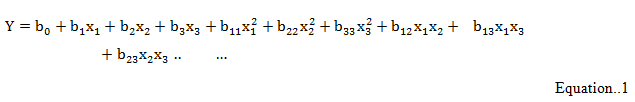Where, Y represents the responses and b0, b1, b2, ………, b23 are the coefficients of the model. The coefficients of main and interaction effects were determined by using the standard method. The response surface equations are calculated for prediction of responses.

8. Optimization of Fabric Properties
The optimum fabric performance was predicted by using equations at all levels of variables drawing contours.

9. Adequacy of Models
The followings terms were studied for the adequacies of the models;

a. Difference (%) is calculated by using the following equationb. Accuracy (%) is calculated by using the following equationc. Discrepancy Factor (R-Factor) is calculated  by using the following equationWhere R= Discrepancy Factor, Pa= Actual Values, Pp = Predicted Values.

d. L-estimation is calculated by using the following equationWhere,Where,3. Results and Discussions
The actual observations, predicted values, different calculated parameters for adequacy, response surface equations, and coefficient of co-relation values are given in Table:2. The respective contours at different level of variables were constructed, are given in Figure:  1.1 to 1.3. The discussions are as follows.

Table: 2 Comparison of Actual and Predicted Values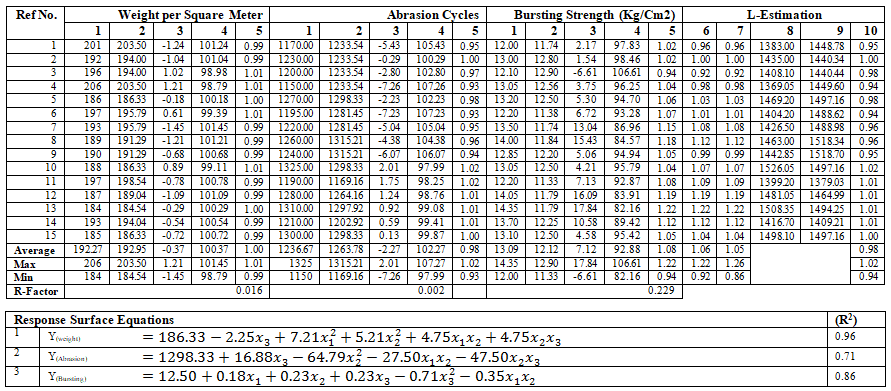1= Actual Value, 2=Predicted Value, 3= Difference (%), 4=Accuracy (%), 5= Actual Values/Predicted Values, 6=Product of 1(all parameters)/Product of 2(all parameters), 7=Product of 5(all parameters), 8= sum of all actual values of parameters, 9= sum of all predicted values of parameters and 10= product (8*9)

Figure: 1. Fabric Performances

Figure: 1. 1.Weight at Different Level of Variables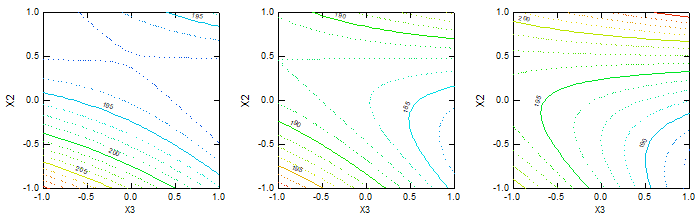(1) Weight at Fiber Length (mm) (a) 65, (b) 70 and (c) 75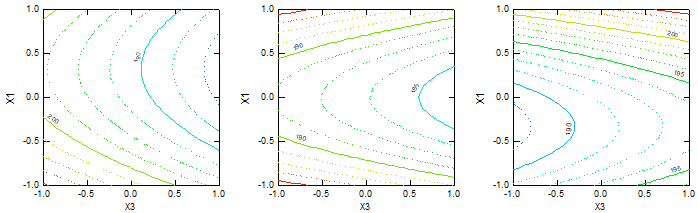(2) Weight at TM (a) 2.00 (b) 2.25 (c) 2.50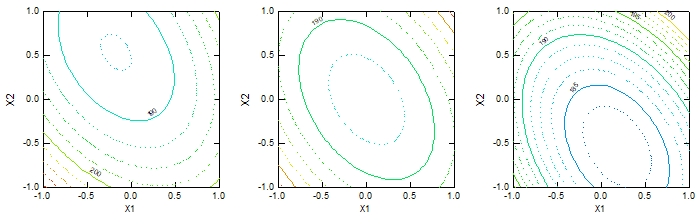(3) Weight at Spacing (mm) (a) 10 (b) 14 (c) 18

Figure: 1. 2. Abrasion at Different Level of Variables(1) Abrasion at Fiber Length (mm) (a) 65, (b) 70 and (c) 75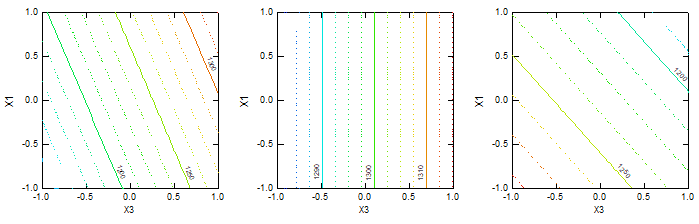(2) Abrasion at TM (a) 2.00 (b) 2.25 (c) 2.50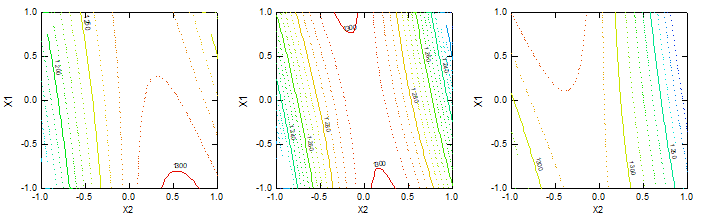(3) Abrasion at Spacing (mm) (a) 10 (b) 14 (c) 18

Figure:1.3 Bursting at Different Level of Variables(1) Bursting at Fiber Length (mm) (a) 65, (b) 70 and (c) 75(2) Bursting at TM (a) 2.00 (b) 2.25 (c) 2.50(3) Bursting at Spacing (mm) (a) 10 (b) 14 (c) 18

3.1 Weight at Different Level of Variables
From Figure: 1.1, It is depicted in (1) (a), TM decreases from 2.25 and spacing increases the weight reduces. The trend is miscellaneous.  (b), the trend is miscellaneous, TM and spacing increases the weight is decreases consistently. (c), up to 14 mm spacing as TM decreases form 2.25 weight decreases however at slightly above to 2.25 TM as spacing increases the weight also increases.

It is depicted in (2) (a), two trends were find, first  increasing weight as spacing increases in fiber length above coded level +0.5, second decreasing as length decreases from 70 mm. (b), as length decreases from 70 mm and spacing increases to 18 mm weight decreases. In length above 70 mm and spacing increases, weight increases. And below reverse trend is visible. (c), in 70 to 75 mm length as spacing increases, weight reduces however when from 65 to 70 mm weight increases.

It is depicted in (3) (a) as TM decreases and length increases up to 70 mm, weight decreases however in further increases in length weight increases. (b), as TM decreases and length up to 70 mm weight decreases however in further increases in length weight increases. (c), optimums were found at TM 2.25 and fiber length 70 mm.

3.2 Abrasion at Different Level of Variables
From Figure: 1.2, It is depicted in (1) (a), The trend were miscellaneous, as at TM up to 2.25 and spacing near to 14 mm optimum is seen. (b), as TM and spacing increases the abrasion cycles are reduced up to certain limit. At TM and spacing level 0 the optimum is found.  (c), the trend was almost similar to (b).

It is depicted in (2) (a), as length and spacing increases abrasion increases. The abrasion cycles are lowest at low fiber length. All parallel lines shows similar trend in all spacing  (b), the parallel horizontal lines shows optimum could not be found in the range and the fiber length increases at all spacing abrasion increases. (c), the trend is almost similar to the (a) as length decreases and spacing increases from the abrasion cycles are decreases. As all lines are parallel shows same relationship at each spacing and length.

It is depicted in (3) (a), as TM increases and length decreases the tendency of reduction in abrasion cycles are noted. (b), as length decreases and TM below 2.25, the abrasion is reduces. After TM 2.25 as length increases abrasion decreases. (c), as the length decreases and TM increases and abrasion is reduces, the trend is miscellaneous.

3.3 Bursting at Different Level of Variables
From Figure: 1.3, It is depicted in (1) (a), as spacing and TM increases up to certain TM bursting increases. (b), as TM increases and spacing decreases at certain point bursting was optimum. Then further increase in TM and decrease in spacing bursting decreases. (c), as TM from 2.25 and spacing from 14 mm increases bursting increases.

It is depicted in (2) (a), as spacing increases below 70 mm length decreasing trend is observed however at above 14 mm spacing trend was reversed. (b), as length and spacing increases the bursting decreases up to 70 mm length. (c), the miscellaneous trends were noted as after 70 mm length and spacing increases the bursting increases also as length increases from 70 mm as spacing increases bursting is decreases.

It is depicted in (3) (a), as TM decreases and fiber length increases up to 70 mm bursting decreases however from 70 to 75 mm length as TM decreases from 2.25 to 2.50 bursting also decreases. (b), as TM decreases up to 2.25 and fiber length increases up to 70 mm bursting increases and further in decreases in TM bursting decreases.  (c), as length increases and TM decrease bursting increases first up to certain length than decreases.

The fabric performance is proportional to the characteristics of fiber, yarn and knit structure. In the study selected variables are mainly impact on yarns and yarns are associated with knitted fabric.

In DRF spinning as fiber length increases more length traps in drafting results, a more compact yarn. At optimum TM, the emerging fibers from front roller nip are trapped at higher binding force and gains better packing density. Due to high packing density weight per unit length of yarn is higher.

In higher spacing, convergence angle is greater, which generates higher false twist as strand results more compact trapping of surface fibers ultimately more compact yarn structure. In DRF production after optimum TM, there is comparatively loosely packed yarn which shows less weight per unit length of fabric. Also after or before optimum spacing between strands, the trapping of fiber reduces cause loose structure of yarn, ultimately as fiber length increases the weight of fabric decreases also the abrasion and bursting strength reduces. In increase of TM and strand spacing up to certain limit (optimum condition of spinning) weight, abrasion and bursting strength increases however after or before optimum condition adverse behaviors are seen. The probable reason may be that up to a certain limit helps in better insertion of twist in single strand which cause better trapping of fibers in the yarn periphery and thereby improvement in various properties of yarn and respective  fabrics.

In other study almost similar findings was reported by R. Ghasemi and other workers that wool/polyester blended worsted yarn is successfully produced by feeding two roving in spinning system and that yarns specifica­tions such as tensile strength, elongation and abrasion resistance are remain almost un-changed.

Literature reveals that as fiber length, TM or strand spacing reaches to the optimum value, the yarn produced are more compact due to better binding. The numbers of fibers in unit length are higher. The numbers of fibers present in the yarn structure are directly proportional to the ultimate product i.e. knitted fabric. The weight of unit area also increases or decreases respectively  due to fiber trappings. The similar is admitted by other researchers that as in same manufacturing conditions the breaking strength, tearing strength, abrasion resistance and crease recovery properties of fabric are improved in case of DRF yarn than plies yarn .

3.4 Adequacy of Models
The comparative analysis between actual and predicted performance of DRF yarn knitted fabric is shown by line diagram as given in Figure:  2.1 to 2.3. The discussions are as follows.

The Figure: 2.1 depict, that trend is almost similar in actual and predicted weight per square meter. The actual weight per square meter were maximum 206, minimum 184 and average 192.27 however predicted weight per square meter were maximum 203.50, minimum 184.54 and average 192.95 respectively.

The Figure: 2.2 depict that abrasion cycles trend of predicted values are different from actual values up to maximum values therefore it remain almost same. The actual abrasion cycles were maximum 1325, minimum 1150 and average 1236.67 however predicted actual cycles were maximum 1315.21, minimum  1169.16 and average 1263.78 respectively.

The Figure: 2.3 depict, that Bursting Strength trend of predicted values are different from actual values. The actual Bursting Strength were maximum 14.35, minimum 12.00 and average  13.09 however predicted Bursting Strength were maximum 12.90, minimum 11.33 and average 12.12 respectively.

The coefficients of correlation (R2) between observed and predicted values were 0.96, 0.71, and 0.86 for weight, abrasion and bursting respectively shows significant influence.

The difference (%) were maximum 1.21, 2.01 and 17.84, minimum were -1.45, -7.26 and -6.61 and average -0.37, -2.27 and 7.12  for weight, abrasion and bursting respectively.

The accuracy  (%) were maximum 101.45, 107.27 and 106.61, minimum were  98.79, 97.99 and 82.16 and average 100.37, 102.27 and 92.88  for weight, abrasion and bursting respectively.

The Discrepancy Factor (R-Factor) was noted 0.016, 0.002 and 0.229 for weight, abrasion and bursting respectively.

The values under L-estimation are as follows:

• The values of ratio were maximum 1.01, 1.02 and 1.22 minimum were 0.99, 0.93 and 0.94 and average1.00, 0.98 and 1.08 for weight, abrasion and bursting respectively.
• The multiple-ratios were calculated maximum 1.26 minimum 0.86 and average 1.05.
• The values of ratio products were calculated maximum 1.22 minimum 0.92 and average 1.06.
• The values of toting ratio were calculated maximum 1.02 minimum 0.94 and average 0.98.

Figure: 2 Comparative Analysis Actual V/s Predicted Performance

4. Conclusions

1. The second-order model is the most-frequently used approximating polynomial model in RSM. The Box–Behnken is most suited design for optimization and prediction of data in textile manufacturing and this model is well-suited for DRF technique yarn knitted fabric.
2. The higher wool fiber length shows higher, fabric weight, abrasion and bursting strength.
3. The correlation of TM is not visible.
4. The role of strands spacing is dominant in comparison to other variables, at 14 mm spacing shows optimum behaviors.
5. The optimum were; weight(gms/mt2) 206 at length 75 mm, TM 2.5 and 14 mm spacing, abrasion(cycles) 1325 at length 70 mm, TM 2.25 and 14 mm spacing and bursting(kg/cm2) 14.35 at length 70 mm, TM 2.00 and 18 mm spacing.
6. The variables have substantial influence.
7. The adequacies of response surface equations are very high.
8. The line trends of knitted fabric basic characteristics were almost same for actual and predicted models.
9. The difference (%) was in range of 1.21 to -1.45, 2.01 to -7.26   and 17.84 to -6.61, the accuracy (%) was in range of 101.45 to 98.79, 107.27 to 97.99 and 106.61 to 82.16 and the Discrepancy Factor (R-Factor) was noted 0.016, 0.002 and 0.229 for weight, abrasion and bursting respectively between actual and predicted data’s.
10. The L-estimation factors for actual and predicted data were i) ratio were in range of 1.01 to 0.99, 1.02 to 0.93 and 1.22 to 0.94 for weight, abrasion and bursting respectively, ii) The multiple-ratio was in range of 1.26 to 0.86, iii) The ratio product was in range of 1.22 to 0.92., iv) The toting ratio was in range of 1.02 to 0.94.

5. References

1. M. Wardrop and R.H. Myers, Some response surface designs for finding optimal conditions, J. Stat. Plann. Infer. 25 (1990), pp. 7-28.
2. E.P. Box and K.B. Wilson, On the experimental attainment of optimum conditions, J. Roy. Stat. Soc. B Stat. Meth. 13 (1951), pp. 1-14.
3. C. Montgomery, Design and Analysis of Experiments: Response surface method and designs, John Wiley and Sons, Inc, New York, (2005).
4. I. Khuri and S. Mukhopadhyay, Response surface methodology, Wiley Interdiscip. Rev. Comput. Stat. 2(2010), pp. 128-149.
5. A. Sakkas, M. Azharul Islam, C. Stalikas, and T.A. Albanis, Photocatalytic degradation using design of experiments: a review and example of the Congo red degradation, J. Hazard. Mater. 175 (2010), pp. 33-44.
6. A. Bezerra, R.E. Santelli, E.P. Oliveira, L.S. Villar, and L.A. Escaleira, Response surface methodology (RSM) as a tool for optimization in analytical chemistry, Talanta 76 (2008), pp. 965-977.
7. H. Doehlert, Uniform shell designs, J. Roy. Stat. Soc. C Appl. Stat. 19 (1970), pp. 231-239.
8. R.T. Tarley, G. Silveira,W.N.L. dos Santos, G.D. Matos, E.G.P. da Silva, M.A. Bezerra, M. Miró, and S.L.C. Ferreira, Chemometric tools in electroanalytical chemistry: methods for optimization based on factorial design and response surface methodology, Microchem. J. 92 (2009), pp. 58-67.
9. E.P. Box and D.W. Behnken, Some new three-level designs for the study of quantitative variables, Technometrics 2 (1960), pp. 455-475.
10. Brown, R. Tauler, and R. Walczak, Comprehensive Chemometrics, Vol. 1, Elsevier, Amsterdam (2009), pp. 345-390.
11. Oughlis-Hammache, N. Hamaidi-Maouche, F. Aissani- Benissad, and S. Bourouina-Bacha, Central composite design for the modeling of the phenol adsorption process in a Fixed-Bed reactor, J. Chem. Eng. Data 55 (2010), pp. 2489-2494.
12. H. Myers and D.C. Montgomery, Response surface methodology, Process and product optimization using designed experiments, John Wiley & Sons, Inc., New York (2002).
13. T. Hoke, Economical second-order designs based on irregular fractions of the 3k factorial, Technometrics 16 (1974), pp. 375-384.
14. J. Box and N.R. Draper, Factorial designs, the |XꞌX| criterion and some related matters, Technometrics 13 (1971), pp. 731-742.
15. G. Roquemore, Hybrid designs for quadratic response surfaces, Technometrics 18 (1976), pp. 419-423.
16. Cochran W G & Cox G M, Experimental Design (Asia Publishing House, India), (1963), pp. 347.
17. Skilling J & Bryan R K, Maximum entropy image reconstruction-General algorithm, Royal Astronomical Society (RAS) Monthly Notices, 211 (1984), pp.111-124.
18. Ghasemi, R. Mozafari-Dana, S. M. Etrati,S. Shaikhzadeh Najar, Comparing the physical properties of produced sirospun and new hybrid solo-siro spun blend wool/polyester worsted yarns, Fibers and Textiles  in Eastern Europe, March, 16, 1 (2008), pp. 66.
19. Dieter Geriche,  The Indian Textile Journal, May, (1995), pp. 102.
20. Bhatnagar S., Salotra K.R. and Kaushik R.C.D., The Indian Textile Journal, July, (1994), pp. 52-53.